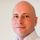cancel
Showing results for
Did you mean:Frequent Visitor

## Calculating a measure that gives me the "right" average ...

Hi,

Earlier I received help form @LivioLanzo to resolve my question on how to structure my data. That worked However ... a new question came up that I cannot seem to resolve. It is about calculating a measure that does NOT do a weighted average 🙂

I want to use this measure in a visualisation so that it shows: (in table form)

 respondent questioncategory question score averagebyquestion averagebyquestioncategory aveargebyrespondent resp1 cat1 cat1.1 5 5 5 4,5 resp1 cat1 cat1.2 5 5 5 4,5 resp1 cat1 cat1.3 5 5 5 4,5 resp1 cat2 cat2.1 5 5 4 4,5 resp1 cat2 cat2.2 3 3 4 4,5

Regardless of the number of questions in a category, each respondent will need to get an average based on the average over all categories. To calculate the total, each category should have one vote. In the example above. The average should be 4,5 as in the table above. I however keep getting 4,6 (the weighted average)

My key problem seems to be how to create a function that iterates not over each row but over a new/filtered? table that contains only the average for a category. Is that at all possible, to use DAX to create a virtual table that contains a subset of rows and columns from the original table as well as the values from a measure?

I even find it difficult to do this in excel so thinking through this problem proves to be harder than I thought it would be - even though the concept seems so straightforward.

Kind regards,

Max

1 ACCEPTED SOLUTIONFrequent Visitor
I thought I solved this question myself - until it turned out I didn't

the path I tried: is to use the formula suggested (the allselected (values version ) to calculate the average for each category and then using that formula inside another calculation that divides the sum of those averages for a respondent by the distinctcount of categories for a respondent. IT came up with the correct average in the total row in the bottom of the table, however I couldn't get it to work on the rows of the table.

Will remain interested in any working and preferably a very elegant approach.&nbsp;

Kind regards,

Max

DAX I ended up trying that got me close but not quite there:

Average Scores := calculate ( average( table[score], filter ( table, table[score] <>0))

Average by respondent := averagex ( values ( questioncategory) , [Average Scores]))

Kind regards,
Max
3 REPLIES 3Community Support

Hi @Max_Kloosterman ,

You can add use allexcept to choose which fields filtered formula calculation.

Sample:

```Count Measure =
CALCULATE (
AVERAGE ( Table[Score] ),
ALLSELECTED ( Table ),
VALUES ( Table[ColumnName] )
)

Count Measure2 =
CALCULATE ( AVERAGE ( Table[Score] ), ALLEXCEPT ( Table, Table[ColumnName] ) )```

Regards,

Xiaoxin Sheng

Community Support Team _ Xiaoxin
If this post helps, please consider accept as solution to help other members find it more quickly.Frequent Visitor

I've tested this solution and it still gives me 4,6 and not 4,5... this is proving to be a hard one. Any other idea's?

To understand it better I've looked at a couple of lessons on The ALL, ALLSELECTED and ALLEXCEPT filters today and am learning more and more. Thanks for that.

One additional question / complication: In my real table respondents are able to score 0 to indicate that they did not want to answer that question. I am trying to figure out where to filter out those values. i.e. where do I insert a filter that ensures only values are considered that fullfil the condition Table[score]<>0? For the interim I have dealt with this by changing all 0 scores to null upon import of the data.

Kind regards,

MaxFrequent Visitor
I thought I solved this question myself - until it turned out I didn't

the path I tried: is to use the formula suggested (the allselected (values version ) to calculate the average for each category and then using that formula inside another calculation that divides the sum of those averages for a respondent by the distinctcount of categories for a respondent. IT came up with the correct average in the total row in the bottom of the table, however I couldn't get it to work on the rows of the table.

Will remain interested in any working and preferably a very elegant approach.&nbsp;

Kind regards,

Max

DAX I ended up trying that got me close but not quite there:

Average Scores := calculate ( average( table[score], filter ( table, table[score] <>0))

Average by respondent := averagex ( values ( questioncategory) , [Average Scores]))

Kind regards,
Max